Select Page

# How Much To Pay?

Financial history has taught us that huge wealth for investors in the market has not been created just by identifying which economy is going to grow at faster rate and not even by identifying which industry is going to gain large market proposition and to be more specific, not even by identifying which company is going to maintain its ‘profitable growth’ nature in future. Even though identifying great asset(company) is important, it is just one part of the equation but rather identifying great company at a great price completes the equation. Financial history has taught us that, paying the right price for the asset has no substitution.

Valuing a business has been and will be an integral part in analyzing the company. In this section, we will be discussing simple elementary valuation framework which can be used by an investor to begin their journey in analyzing and valuing the company.

## A Few Words On Traditional Metrics

Investment community uses various traditional valuation metrics such as ‘Price to Earnings’(P/E), ‘Price to Book’(P/B), ‘Price to Earnings Growth’(PEG) and lot more to quickly identify the ‘valuation’ of the business but these valuation metrics are just valuation shorthand and they come with many ‘blind spots’, these blind spots create more distortion in taking long-term decisions.

## So, how to overcome it?

Instead of devising some new valuation metric, we will be learning two simple frameworks which can be used effectively to get ahead in our analysis. (framework can also be understood even if you have zero knowledge in finance)

### Valuation Frameworks

1. Break-even analysis.
2. Probabilistic Valuation

Both the valuation framework are interconnected with each other and we will see how this interconnection works.

### Break-Even Analysis

First, let us understand ‘What does break-even means’ through a simple example.

Let us consider a company which generates annual revenue of 100 crores and a net profit of 5 crores and now we decide to buy the whole company for 25 crores. Since we have invested 25 crores in the business, we are interested in knowing how long business will take to generate the profit such that the cumulative total profit made by the company greater than the original amount invested.

In our case assuming that the company generates a net profit of 5 crores each year for next five years, it means that at the end of 5 years the ‘total accumulated profit’ is 25 crores. Thus, we can say that, 5 years is the break-even time, it also tells us that, it will take 5 years to get back our original capital (25 crores) if we invest in this business.

Now let us consider more sophisticated example,

A company of Market capitalization of 120 crores generates annual revenue of 100 crores and the net profit of 20 crores and is expected to grow its net profit and revenue at 10% CAGR for the next five years. Calculate the break-even time. Since market capitalization is 120 crores, it means the total amount needed to buy the whole company is 140 crores. So, now we want to find, a total number of years, that the company would take to generate ‘total accumulated’ profit greater than 120 crores.

The table below shows the estimation we get if we simulate our initial condition. From the table, we can easily say that the break-even time is 4 years (box highlighted) because, at the end of the fourth year, total cumulative profit (123 crores) is greater than the total profit (120 crores).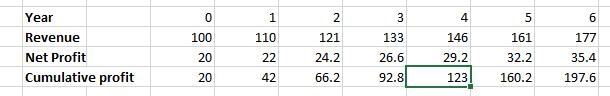## Now we will introduce the concept of ‘Time Value Of Money’ (TVM)

‘Time Value Of Money’ is the idea that, the money that is available at the present time is worth more than the same amount in the future. In our example we have estimated that the break-even point 4 years, that is, we recover our original capital of 120 crores in 4 years but 120 crores is worth more than 120 crores in 4 years from now.

### How to calculate TVM ?

FV = P((1+(r/100)^n)

FV – Future Value (this is the value we have to calculate)

P  – Present Money (in our case 120 crores)

• – Number of years (in our case, it is ‘break-even’ time)

r    – Rate of return (in our case it is risk-freerate’)

### Why To Use Risk Free Rate

‘Risk free Rate’ indicates the rate of return to our initial investments without any substantial risk involved in it, unlike equity investment which involves lots of risk, so if we make any investments in equity, we should demand premium return than 7.79% (current ‘risk free rate’) and if any equity investments give us less than 7.79%, it is considered to a worthless investment.

So again, calculating break-even point by keeping TMV in hand we get the below table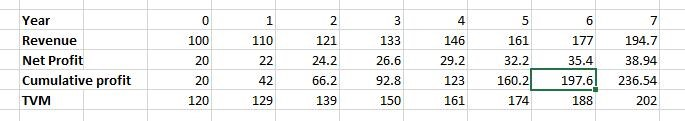Our initial capital 120 crores have grown to 188 crores in six years when adjusted to the time value of money. So, from the table, we get that, at the end of the 6 years, the cumulative profit (197.6 crores) is greater than TVM (188 crores). Thus, we can conclude that our break-even time is 6 years. If we observe more closely, we might see that the break-even time was just 4 years (without considering TVM) and it is 6 years (with considering TMV).

This indicates that ‘thoughtful valuation’ must consider TMV in a valuation of the business.

## Why Should Investor Care About ‘Break-even’ time?

If the calculated break-even time is less than 5-7 years, then it is considered to be a good investment and if the break-even time is higher than 10-15 years, then investments should be avoided.

### So why 5-7 years only?

We have to very conservative while calculating the break-even point because it is very difficult to calculate how much the profit the company is going generate in very long run (10-15 years because the company may have gone even extinct in this time frame) due to various uncertainty and also if break-even point is 10-15 years, one has to wait 10-15 years to recover his original money and top of it TVM also increases a lot.

But unfortunately, we cannot find many good investments, having a break-even point less than 5-7 years in the current market. But by having a ‘watchlist d of investments will help the investors to take advantage of the falling market.

## Probabilistic Valuation

Business works in uncertain environments so, it would be clever to think about the future in ‘range of possibilities’ instead of defining accurate numbers.

In our example, in break-even analysis, we have assumed that company would grow its net profit by 10% CAGR for next five years, but instead of defining a specific number we can mention different outcomes and their probability associated with that outcomes.

For example, instead of saying that company is going to grow its net profit by 15% CAGR for the next five years, we can say that, the company can grow at 15% CAGR and the probability for this happening is 35% and has a probability of 45% for growing at 10% CAGR and has the probability of 20% for growing at 20% CAGR for next five year.

To summarize, see below table,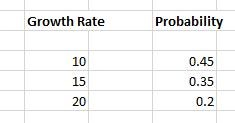## Now we will introduce the concept of ‘Expected value’

Consider we are playing a game called unbiased coin toss (the probability of getting heads or tails in a given coin toss is ½ or 0.5). Now the game is that we gain Rs 5 if heads face up and loss Rs 2 if tails face up during the coin toss). If we play the game for 10 rounds, what is the ‘expected money’ we can get at the end of the game?

Expected value(money) can be calculated by using the below formula:

Expected value = (Gain* Probability of gain) + (Loss*Probability of loss)

So as per our example, EV = ( +5 * 0.5 ) + ( -2 *0.5) = 1, so the expected money we may earn at the end of one round is Rs 1, so at the end of 10 rounds we may earn Rs 10 ( 10 * Rs1). Now we can conclude by saying that, the expected money one can earn at the end of 10 rounds will be Rs 10. Coming back to our probabilistic valuation, since we are approaching the future growth rate of the business in ‘range of probabilities’ it is necessary to calculate our expected value.

Now we can calculate of expected value as per our example,We get our expected growth rate to be 13.75% CAGR. Now we can take this growth rate as 13.75% as the base rate and construct the break-even analysis described in our first framework and calculate the ‘break-even’ time.

## Limitations and Mitigations:

### Limitations:

‘Probabilistic valuation’ framework would provide more accurate result than the former framework but the main problem is:

• How many ranges of outcomes should we consider?
• How to assign the probability to those outcomes?

### Mitigation:

‘Ranges of outcomes’

• It is necessary to have at least 3 probable outcomes (low, medium and high) and a number of outcomes should not be increased for the sake of adding new outcomes because it dilutes the essence of probability.
• The ranges of outcomes should be updated periodically over a period of time as we get new information.

‘Assigning Probability’

• Assigning probability is the trickiest part in this process so, one should be more conservative in assigning the probability and one should not get biased on one particular outcome.

• One should periodically update these probabilities as we get new information regarding the particular outcome.

### For example:

If we get the information that the company is going to expand its business and start producing products next year, we should increase the probability of our ‘high growth rate’ and on the other side if we get the information that the company is going to ‘shut down’ its plants, we must increase the probability of ‘lower growth rate’.

In this section, we have discussed in-detail of two broad elementary framework which is just our first step taken by us in analysis and this framework should be used with many other valuation frameworks such as DCF, free cash flow etc to get better insights about the valuation of the company.

## A Quick Question

The market capitalization of TCS is Rs 6.8 lakh crores and produces net profit is Rs 28759 crores. Now consider the table below: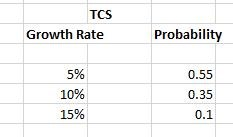• Calculate the ‘Break-even’ time.

• Now consider that, if the investor wants to earn 15% CAGR for the next five years, find if TCS is suitable for his investments or not? (this question is quite tricky but if you understood the above concept completely you can answer this).

Answers for both the questions will be shared in the upcoming post so, stay tuned.

### It is your turn

What do you think? Will this really help you or your friend and communities? Share your views under the comment section.

### Wait before you go.... People of all ages discover something they didn’t expect. Share this to stay connected with your friends and community.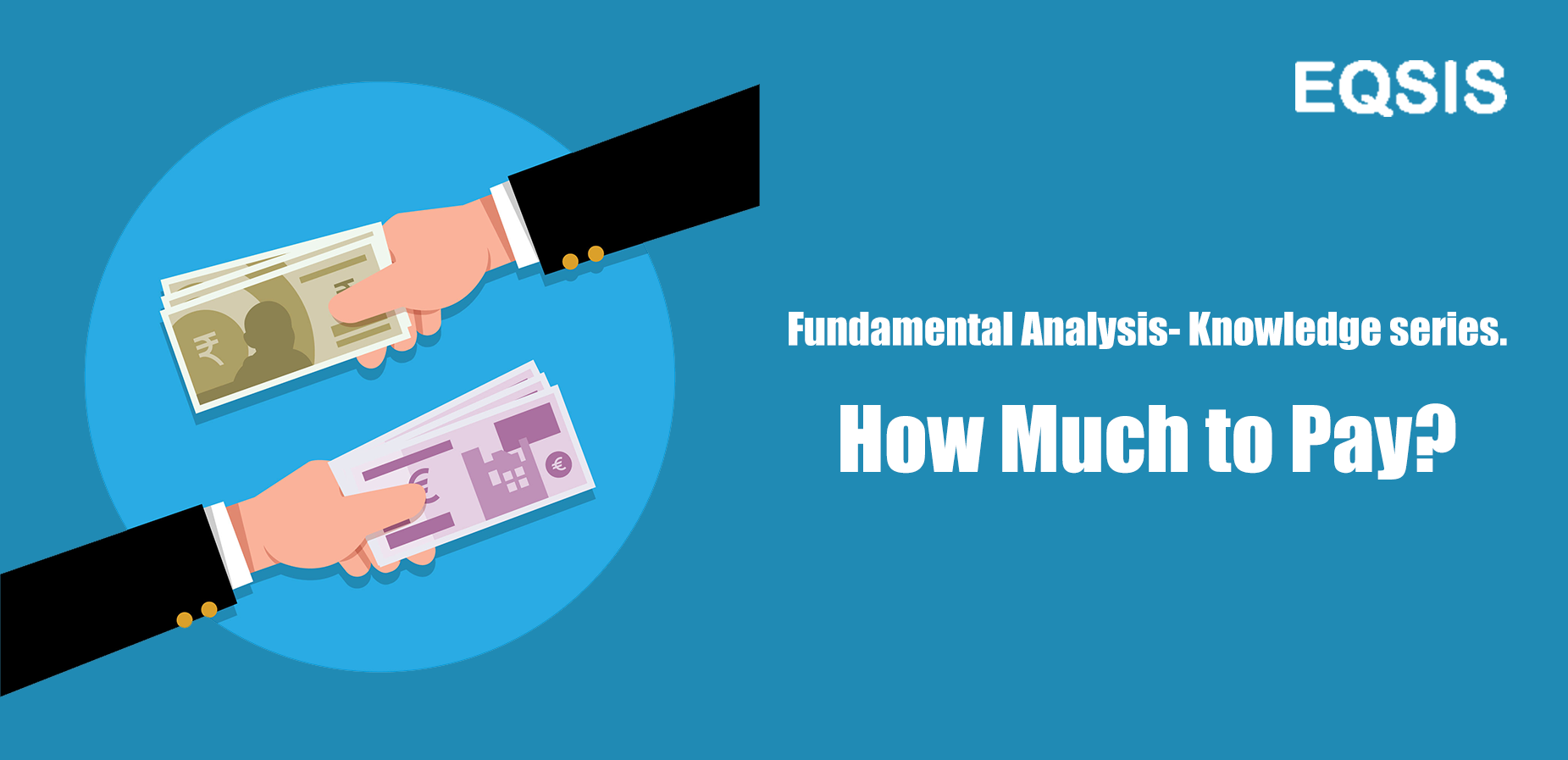## How Much To Pay?

How Much To Pay? Financial history has taught us that huge wealth for investors in the market has not been created just by identifying which economy is going to grow at faster rate and not even by identifying which industry is going to gain large market proposition and to be more specific, not even by identifying which company is going to maintain its ‘profitable growth’ nature in future. Even though identifying great asset(company) is important, it is just one part of the equation but rather identifying great company at a great price completes the equation. Financial history has taught us